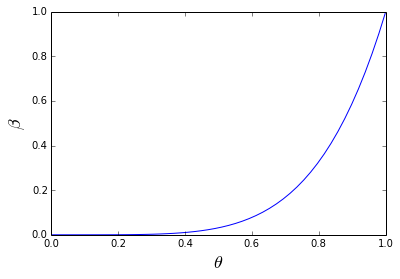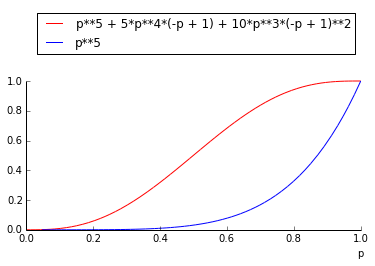In :
%matplotlib inline


Given the coin-tossing experiment with unknown parameter $p$. The individual coin-flips are Bernoulli distributed. As we discussed previously, the estimator for $p$ is the following:

$\hat{p} = \frac{1}{n} \sum_{i=1}^n X_i$

We have to separate hypotheses: $H_0$ is the so-called null hypothesis. In our case this can be

$$H_0 : p \lt \frac{1}{2}$$

and the alternative hypothesis is then

$$H_1 : p \ge \frac{1}{2}$$

To choose between these, we need a statistical test that is a function, $G$, of the sample set $\mathbf{X}_n=\left\{ X_i \right\}_n$. This test will have a threshold, something like

$$G(\mathbf{X}_n) < c \implies H_0$$

and otherwise choose $H_1$. To sum up at this point, we have the observed data $\mathbf{X}_n$ and a function $G$ that will somehow map that data onto the real line. Then, using the constant $c$ as a threshold, the inequality effectively divides the real line into two parts, one corresponding to each of the hypotheses.

Whatever this test $G$ is, it will make mistakes of two types -- false negatives and false positives. The false positives arise from the case where we declare $H_0$ when the test says we should declare $H_1$. Here are the false positives (i.e. false alarm):

$$\mathbb{P}_{FA} = \mathbb{P}( G(\mathbf{X}_n) \gt c \vert p \le \frac{1}{2})$$

Or, alternatively,

$$\mathbb{P}_{FA} = \mathbb{P}( G(\mathbf{X}_n) \gt c \vert H_0)$$

Likewise, the other error is a false negative, which we can write analogously as

$$\mathbb{P}_{FN} = \mathbb{P}( G(\mathbf{X}_n) \lt c \vert H_1)$$

Thus, by choosing some acceptable values for either of these errors, we can solve for the other one. Later we will see that this is because these two quantities are co-dependent. The practice is usually to pick a value of $\mathbb{P}_{FA}$ and then find the corresponding value of $\mathbb{P}_{FN}$. Note that it is traditional to speak about detection probability, which is defined as

$$\mathbb{P}_{D} = 1- \mathbb{P}_{FN} = \mathbb{P}( G(\mathbf{X}_n) \gt c \vert H_1)$$

In other words, this is the probability of declaring $H_1$ when the test exceeds the threshold. This is Otherwise known as the probability of a true detection.

## Back to the coin flipping example¶

In our previous problem, we wanted to derive an estimator for the underlying probability for the coin flipping experiment. For this example, we want to ask a softer question: is the underlying probability greater or less than 1/2. So, this leads to the two following hypotheses:

$$H_0 : p \lt \frac{1}{2}$$

versus,

$$H_1 : p \ge \frac{1}{2}$$

5 Let's suppose we want to determine is based upon five observations. Now the only ingredient we're missing is the $G$ function and a way to pick between the two hypotheses. Out of the clear blue sky, let's pick the number of heads observed in the sequence of five observations. Thus, we have

$$G (\mathbf{X}_5) := \sum_{i=1}^5 X_i$$

and, suppose further that we pick $H_1$ only if exactly five out of five observations are heads. We'll call this the all-heads test.

Now, because all of the $X_i$ are random variables, so is $G$. Now, to find the corresponding probability mass function for this. As usual, assuming independence, the probability of five heads is $p^5$. This means that the probability of rejecting the $H_0$ hypothesis (and choosing $H_1$, because there are only two choices here) based on the unknown underlying probability is $p^5$. In the parlance, this is known and the power function as in denoted by $\beta$ as in

$$\beta(\theta) = \theta^5$$

where I'm using the standard $\theta$ symbol to represent the underlying parameter ($p$ in this case). Let's get a quick plot this below.

In :
px = linspace(0,1,50)
plot( px, px**5)
xlabel(r'$\theta$',fontsize=18)
ylabel(r'$\beta$',fontsize=18)

Out:
<matplotlib.text.Text at 0x76eb790>Now, to calculate the

$$\mathbb{P}_{FA} = \mathbb{P}( G(\mathbf{X}_n)= 5 \vert H_0)$$

or, in other words,

$$\mathbb{P}_{FA}(\theta) = \mathbb{P}( \theta^5 \vert H_0)$$

Notice that this is a function of $\theta$, which means there are many false alarm probability values that correspond to this test. To be on the conservative side, we'll pick the maximum (or, supremum if there are limits involved) of this function, which is known as the size of the test, traditionally denoted by $\alpha$.

$$\alpha = \sup_{\theta \in \Theta_0} \beta(\theta)$$

which in our case is

$$\alpha = \sup_{\theta < \frac{1}{2}} \theta^5 = (\frac{1}{2})^5 = 0.03125$$

which is a nice, low value. Likewise, for the detection probability,

$$\mathbb{P}_{D}(\theta) = \mathbb{P}( \theta^5 \vert H_1)$$

which is again a function of the parameter $\theta$. The problem with this test is that the $\mathbb{P}_{D}$ is pretty low for most of the domain of $\theta$. Useful values for $\mathbb{P}_{D}$ are usually in the nineties, which only happens here when $\theta \gt 0.98$. Ideally, we want a test that is zero for the domain corresponding to $H_0$ (i.e. $\Theta_0$) and equal to one otherwise. Unfortunately, even if we increase the length of the observed sequence, we cannot escape this effect with this test. You can try plotting $\theta^n$ for larger and larger values of $n$ to see this.

### Majority Vote Test¶

Due to the problems with the detection probability we uncovered in the last example, maybe we can think of another test that will have the performance we want. Suppose we reject $H_0$ if the majority (i.e. more than half) of the observations are heads. Then, using the same reasoning as above, we have

$$\beta(\theta) = \sum_{k=3}^5 \binom{5}{k} \theta^k(1-\theta)^{5-k}$$

Using some tools from sympy, we can plot this out and compare it to the previous case as in the cell below.

In :
from sympy.abc import p,k # get some variable symbols
import sympy as S

expr=S.Sum(S.binomial(5,k)*p**(k)*(1-p)**(5-k),(k,3,5)).doit()
p0=S.plot(p**5,(p,0,1),xlabel='p',show=False,line_color='b')
p1=S.plot(expr,(p,0,1),xlabel='p',show=False,line_color='r',legend=True,ylim=(0,1.5))
p1.append(p0)
p1.show()In this case, the new test has size

$$\alpha = \sup_{\theta < \frac{1}{2}} \theta^{5} + 5 \theta^{4} \left(- \theta + 1\right) + 10 \theta^{3} \left(- \theta + 1\right)^{2} = (\frac{1}{2})^5 = 0.5$$

which is a pretty bad false alarm probability. Values less that one percent are usually considered acceptable. As before we only get to upwards of 90% for detection probability only when the underlying parameter $\theta > 0.75$. As before, this is not so good. Let's run some simulations to see how this plays out.

In :
# implement 1st test where all five must be heads to declare underlying parameter > 0.5
from scipy import stats
b = stats.bernoulli(0.8) # true parameter is 0.8 (i.e. hypothesis H_1)
samples = b.rvs(1000).reshape(-1,5) # -1 means let numpy figure out the other dimension i.e. (200,5)
print 'prob of detection = %0.3f'%mean(samples.sum(axis=1)==5) # approx 0.8**3

prob of detection = 0.405

In :
# here's the false alarm case
b = stats.bernoulli(0.3) # true parameter is 0.3 (i.e. hypothesis H_0)
samples = b.rvs(1000).reshape(-1,5)
print 'prob of false alarm = %0.3f'%mean(samples.sum(axis=1)==5)

prob of false alarm = 0.000


The above two cells shows that the false alarm probability is great, but the detection probability is poor. Let's try the same simulation for the majority vote test.

### Majority Vote Test¶

In :
# implement majority vote test where three of five must be heads to declare underlying parameter > 0.5
b = stats.bernoulli(0.8) # true parameter is 0.8 (i.e. hypothesis H_1)
samples = b.rvs(1000).reshape(-1,5)
print 'prob of detection = %0.3f'%mean(samples.sum(axis=1)>=3)

prob of detection = 0.935

In :
# here's the false alarm case
b = stats.bernoulli(0.3) # true parameter is 0.3 which means it's hypothesis H_0
samples = b.rvs(1000).reshape(-1,5)
print 'prob of false alarm = %0.3f'%mean(samples.sum(axis=1)>=3)

prob of false alarm = 0.165


Both of the simulation results follow our earlier analysis. Try tweaking the underlying parameter (i.e. 0.8, 0.3) here and see how the simulation reacts. Not surprisingly, the majority vote test does better when the underlying parameter is much greater than 0.5

## P-Values¶

As we have seen there are a lot of moving parts in hypothesis testing. What we need is a simple way to statistically report the findings. The idea is that we want to find the minimum level at which the test rejects $H_0$. Thus, the p-value is the probability, under $H_0$, that the test-statistic is at least as extreme as what was actually observed. Informally, this means that smaller values imply that $H_0$ should be rejected, although this doesn't mean that large values imply that $H_0$ should be retained. This is because a large p-value can arise from either $H_0$ being true or the test having low statistical power.

If $H_0$ happens to be true, the p-value is like a uniformly random draw from the interval $(0,1)$. If $H_1$ is true, the distribution of the p-value will concentrate closer to zero. For continuous distributions, this can be proven rigorously and implies that if we reject $H_0$ when the corresponding p-value is less than $\alpha$, then the probability of a false alarm (a.k.a. type I error) is $\alpha$. Perhaps it helps to formalize this a bit before we get to computing it. Suppose $\tau(X)$ is a test statistic that rejects $H_0$ as it gets bigger. Then, for each sample $x$, corresponding to the data we actually have on-hand, we define

$$p(x) = \sup_{\theta \in \Theta_0} \mathbb{P}_{\theta}(\tau(X) \ge \tau(x))$$

Here's one way to think about this. Suppose you developed a really controversial study and you are ready to report your results, and someone says that you just got lucky and somehow just drew data that happened to correspond to a rejection of $H_0$. What do you do? In a perfect world, someone else would replicate your study (thereby obtaining a different draw) and hopefully also reject $H_0$ just as you did. What p-values provide is a way to addressing this by capturing the odds of just a favorable data-draw. Thus, suppose that your p-value is 0.05. Then, what you are showing is that the odds of just drawing that data sample, given $H_0$ is in force, is just 5%. This means that there's a 5% chance that you somehow lucked out and got a favorable draw of data.

Let's make this concrete with an example. Given, the majority-vote rule above, suppose we actually do observe three of five heads. Given the $H_0$, the probability of observing this event is the following:

$$p(x) =\sup_{\theta \in \Theta_0} \sum_{k=3}^5\binom{5}{k} \theta^k(1-\theta)^{5-k} = \frac{1}{2}$$

For the all-heads test, the corresponding computation is the following:

$$p(x) =\sup_{\theta \in \Theta_0} \theta^5 = \frac{1}{2^5} = 0.03125$$

From just looking at these p-values, you might get the feeling that the second test is better, but we still have the same detection probability issues we discussed above; so, p-values help in summarizing and addressing aspects of our hypothesis testing, but they do not summarize all the salient aspects of the entire situation.

## Summary¶

In this section, we discussed the structure of statistical hypothesis testing and defined the various terms that are commonly used for this process, along with the illustrations of what they mean in our running coin-flipping example. From an engineering standpoint, hypothesis testing is not as common as confidence-intervals and point estimates, which we will discuss shortly. On the other hand, hypothesis testing is very common in social and medical science, where one must deal with practical constraints that may limit the sample size or other aspects of the hypothesis testing rubric. In engineering, we can usually have much more control over the samples and models we employ because they are typically inanimate objects that can be measured repeatedly and consistently. This is obviously not so with human studies, which generally have many more confounding factors. Nevertheless, as we will see, hypothesis testing can provide another useful tool for examining our own model assumptions within an engineering context.